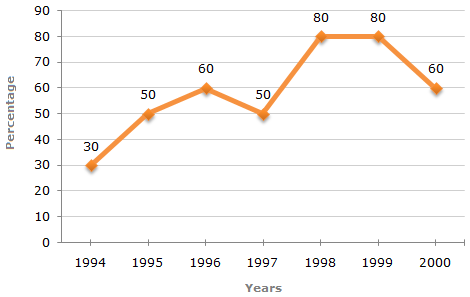# Data Interpretation - Line Charts - Discussion

### Discussion :: Line Charts - Line Chart 5 (Q.No.2)

The following line graph gives the percentage of the number of candidates who qualified an examination out of the total number of candidates who appeared for the examination over a period of seven years from 1994 to 2000.

Percentage of Candidates Qualified to Appeared in an Examination Over the Years2.

In which pair of years was the number of candidates qualified, the same?

 [A]. 1995 and 1997 [B]. 1995 and 2000 [C]. 1998 and 1999 [D]. Data inadequate

Explanation:

The graph gives the data for the percentage of candidates qualified to appeared and unless the absolute values of number of candidates qualified or candidates appeared is know we cannot compare the absolute values for any two years.

Hence, the data is inadequate to solve this question.

 Jyoti said: (Jun 3, 2014) In this problem, we have the same of number students appeared for the examination for the all seven years i.e. we have the same number students for 1998 and 1999 because we are taking it for the whole seven years. Then in this we have 80% of total of students in both the cases. Then how can we say that we don't have the proper data.

 D.P said: (Feb 4, 2017) Here, the number of students appeared for the examination is may be different or not. So we can't decide. Therefore, answer data is inadequate.

 Dhananjoyreang said: (Sep 6, 2017) I can't understand. Explain.

 Deepti said: (Feb 18, 2018) The graph here is mentioning about the percentage of students qualified but not the students who appeared. Since we do not know the number of students appeared for the exam over the period of seven years so the answer will be D.